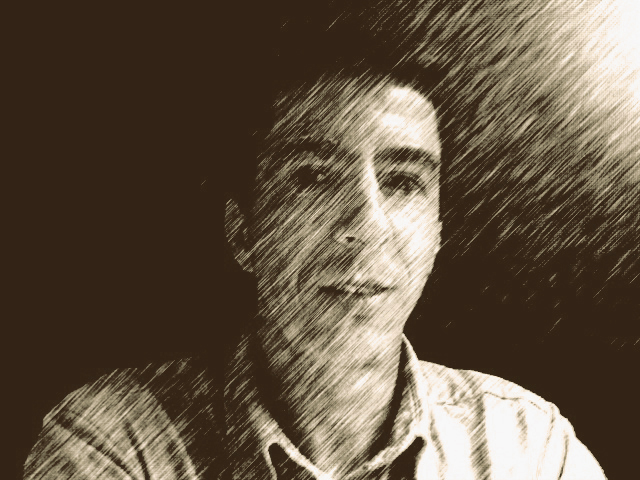Stephen P. Shipman Professor Department of Mathematics Louisiana State University» Home
» Research
» Courses
 4200 F 2019 7320 S 2019 7384 S 2019 7311 F 2015 7384 S 2014 2030 F 2013 VIR F 2012 7384 S 2012 7380 F 2009
» REU
» Funding
» Contact

Math Department
Am. Math Soc.
Math. Assoc. Am.
Nat. Sci. Found.

Barbara
Michael
Patrick

Art

Birds

## Partial Differential Equations

### Prof. Stephen Shipman

Place: Room 130 of Lockett Hall
Time: Tuesday and Thursday from 1:40 to 3:00

Office: Room 314 of Lockett Hall
Telephone: 225/578-1674
Email: shipman@math.lsu.edu
Office Hours: Monday 9:00-11:00 and Wednesday 1:00-3:00 or by appointment

## Course Synopsis

The aims of the course are these:

To provide an overview of the diversity of mathematics and applications subsumed by or connected to partial differential equations.

To delve into the theory of some specific topics.

To impart a necessary intuition for PDEs, specifically for those of mathematical physics. This entails delving into the details of specific examples.

To learn techniques and theory from hard analysis and abstract functional analysis that are necessary tools for analyzing PDEs. Most of the time all the details of the theory we use can be provided. At times we will have to use a theorem without proof, such as the spectral theorem; in these cases, we will understand the significance and application of the theory and give references to its development.

Here is a list of topics that I would like to discuss, not necessarily in the order given. We will definitely try to do the first eight topics in some capacity.

Basic linear equations and separation of variables: the diffusion equation, the wave equation, and the Laplace and Poisson equations. Fundamental solutions.

Elliptic equations. Weak formulation of PDEs and the associated abstract functional analysis; applications to materials science.

Unitary groups, and the role of self-adjoint extensions of symmetric operators in boundary-value problems.

Fourier transform methods.

Waves. Nonlinear and linear waves, dispersion relations, hyperbolic systems and shocks; the Korteweg-deVries equation.

Asymptotic analysis: the method of stationary phase and WKB theory; application to the vanishing-dispersion limit of the KdV equation.

The method of characteristics for first-order equations.

Calculus of variations, the Hamilton-Jacobi equation, the iconal equation in geometric optics.

Boundary-integral equations and their connection to complex variables and wave scattering.

Special relativity: differential geometry and the special role of the wave equation.

Quantization and the Schrödinger equation.

### Literature

We will not use any one source as a text book. My lectures will draw from my notes and several references, including the books of P. R. Garabedian, L. C. Evans, E. C. Titchmarsh, G. B. Whitham, F. Treves, F. John, R. Haberman, and J.-C. Nédélec and the functional analysis books of M. Reed and B. Simon.

There is a bibliography of relevant works below, with links to some PDF files of excerpts.

### Assignments

I will assign problems regularly. At the end of the course, I will give a longer and somewhat comprehensive set of problems that will serve as an out-of-class final exam.

Students may discuss problems with each other and other people (including me, of course) and consult other literature; in fact students are encouraged to search the literature and discuss ideas. However, all work that is turned in must ultimately be that of the submitter alone. If a student receives aid on an assigned problem from discussions with people or other sources, he or she must begin from scratch in writing the solution so that the result is the product of his or her own understanding alone.

### Evaluation

Evaluation of performance in the course is based on performance on the regular assignments and the out-of-class final exam, according the the following weighting:

Regular assignments: 75%
Final exam: 25%

### Lecture Notes and Problem Sets

Notes 1 (pdf)
Notes 2
Notes 3
Notes 4
Notes 5
Notes 6
Notes 7
Notes 8
Notes 9
Notes 10
Notes 11
Notes 12

Problem Set 1 (pdf)
Problem Set 2
Problem Set 3
Problem Set 5

Final Exam

Due dateProblems to do
Thurs., Sept. 3 Problem Set 1
Tues., Sept. 22 Problem Set 2
Thurs., Oct. 22 Problem Set 3
Thurs., Nov. 5 From Haberman, Ch. 7: Problems 7.3.2 and 7.8.2
Thurs., Dec. 3 Problem Set 5
Sat., Dec. 12 Final Exam

### Bibliography

1. Excerpts on compact operators:
Naylor and Sell
Kress
Reed and Simon
Riesz and Sz.-Nagy
Akhiezer and Glazman, Chapter V; see the link below.
2. Michael Reed and Barry Simon, Methods of Modern Mathematics: Vol. I Functional Analysis, Vol. II Fourier Analysis and Self-Adjointness, Vol. III Scattering Theory, Vol. IV Analysis of Operators, excerpts from Vol. I (spectral theorem), Vol. II (self-adjoint extensions), Academic Press, 1980.
3. Gerald B. Folland, Introduction to Partial Differential Equations, Second Edition, Princeton University Press, 1995. Excerpt: Chapter 0.
4. J. Billingham and A. C. King, Wave Motion, Cambridge Texts in Appl. Math., Camb. Univ. Press, 2000. Excerpts: Chapter 7, KdV images.
5. E. A. Coddington and N. Levinson, Theory of Ordinary Differential Equations, McGraw-Hill, 1955. Excerpt: Dependence on initial conditions.
6. Lawrence C. Evans, Partial Differential Equations, AMS Graduate Studies in Math., Vol. 19, 1998.
7. G. B. Whitham, Linear and Nonlinear Waves, Wiley-Interscience, 1973. Excerpt: Burgers' Equation.
8. Fritz John, Partial Differential Equations, Springer-Verlag, Appl. Math. Sci. Vol. 1, Fourth Ed., 1982.
9. Carl M. Bender and Steven A. Orszag, Advanced Mathematical Methods for Scientists and Engineers I: Asymptotic Methods and Perturbation Theory, Springer-Verlag, 1999. Excerpt: Lapace Integrals.
10. Richard Haberman, Applied Partial Differential Equations, Fourth Edition, Pearson Prentice Hall, 2004. Excerpts: Separation of Variables, Waves and the Korteweg-deVries Equation.
11. N. I. Akhiezer and I. M. Glazman, Theory of Linear Operators in Hilbert Space, Dover, 1993, replication of the edition of F. Ungar Publishing, NY, 1961, 1963.
 x@lsu.edu (x=shipman)  Privacy Statement here.  Provide Website Feedback here.  Accessibility Statement here.